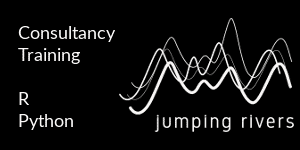GitHub

# Our Logo In R

Published: February 1, 2018Hi all, so given our logo here at Jumping Rivers is a set of lines designed to look like a Gaussian Process, we thought it would be a neat idea to recreate this image in R. To do so we’re going to need a couple packages. We do the usual `install.packages()` dance (remember this step can be performed in parallel).

``````install.packages(c("ggplot2", "ggalt", "readr"))
``````

We’re also going to need the data containing the points for the lines and which set of points belongs to which line. There is a Gist available to download via Jumping Rivers. To read in the CSV file we’re going to use the raw data link.

``````(dd = readr::read_csv("https://goo.gl/HzNbAp", col_types = "ddc"))
## # A tibble: 40 x 3
##       x     y type
##   <dbl> <dbl> <chr>
## 1  36.9  -311 1
## 2  67.9  -332 1
## 3 179    -156 1
## 4 254    -259 1
## # ... with 36 more rows
``````

The data set contains three columns, `x`, `y` and `type`, where `type` indicates the line. Let’s start with a standard `geom_line()`

``````library("ggplot2")
g = ggplot(dd, aes(x, y))
g + geom_line(aes(group = type))
``````The graph shares similarities with our logo, but is too discrete. To smooth the curve, we’ll use a function from the {ggalt} package

``````library("ggalt")
g + geom_xspline(aes(group = type), spline_shape = -0.3)
``````The function `geom_xspline()` is the X-spline version of `geom_line()`, drawing a curve relative to the observations. The parameter`spline_shape = -0.3` controls the shape of the spline relative to the observations. This can be a number between `-1` & `1`. Basically, `-1` = bumpy lines, `1` = flat lines.

Next we’ll change the widths of the lines

``````(g1 = g  + geom_xspline(aes(size = type, group = type), spline_shape = -0.3) +
scale_size_manual(values = (4:1)/2, guide = FALSE))
``````The `scale_size_manual()` function enables us to control the line widths. Finally, we remove the background

``````g1 + theme_void()
``````The function `theme_void()` does what it says on the tin it gives us a theme completely void of everything. Bar the lines of course.

That’s all for now. Thanks for reading! :)